# June, 2016Archive

Jun 20

## Rubbings as Projections

Posted in Uncategorized,

### Overview

This activity introduces children to the abstract concept of projections from 3 dimensional space onto 2 dimensional space in a concrete and age appropriate way by using rubbings of objects with interesting surfaces.  The activity is written for a Kindergarten class, but could easily be adjusted for other early elementary students.    The activity takes 30-50 minutes.  The main portion of the directions are below, but the full version is here: Rubbings as Projections (including the coin equation handout).

### Large group Introduction

1. Demonstrate how to take a rubbing (I used the bottom of my shoe)
2. Discuss what information is preserved in the rubbing (tallest portions) and what is lost (color, how far down the lower parts are)
3. Discuss three dimensions versus two dimensions. If you can only see one side of something, say the top, you don’t know very much about it.  Cone upside down looks the same as a cylinder from the top.  Cube and rectangular prism look the same from the top (if the rectangular prism is oriented correctly).  If you can only see my back, you don’t know how many buttons my shirt has.
4. (optional) Show an object, have students predict what rubbing will look like, take the rubbing and discuss (possibly do this two or three times)
5. Get out the matching cards (RubbingsAsProjections-MatchingCards). Pass out the pictures of the rubbings to groups of students Show the pictures of the objects one at a time on the Elmo/smart board/by holding them up.  Students should try to pick out the picture of the rubbing that goes with the object.  Here’s a sample pair:

### Small group “centers” done in rotation (pick four to do-I think the first four are the best)

1. Doing Rubbings-each student should make a rubbing of the bottom of their shoe.
2. Tallest Portions-cut off and look at the tallest portions of Swiss Cheese, an apple, carrot with the greens still attached, angel food cake, loaf of bread, pear, or similar food objects. Draw a picture.  (“Remember a rubbing is basically taking the top layer of something, so at this station we are going to cut off the top layer of some food.” First I cut the top off the apple (using a kiddie safety knife), “what does this top layer tell us about the rest of it?”  Then used the corer slicer to cut the apple into 3 wedges which the students ate.  Then I help up a small piece of the carrot leaves.  “What do you think this is the top layer of?”  Students knew it was a plant, but didn’t know which one.  Then I got the carrot out of my bag and talked about how the top layer was very different from the main part. Some students wanted to try the carrot too.  For the Swiss cheese I used a package of thin sandwich slices, which I carefully stacked up into the original block they would have come from.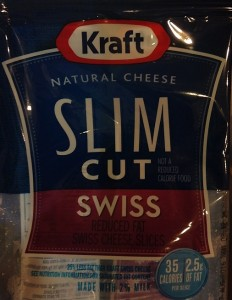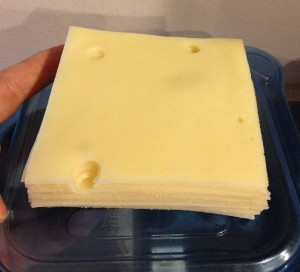“What would it look like if I cut off the top layer of this?”  “Is this more like the apple or the carrot?”  Students also wanted to try the Swiss cheese.  I gave each only a quarter of a slice.  Many students didn’t like it, so I said to spit it out and congratulated them for trying something knew).
3. Memory- Play concentration/memory with the matching cards. The matched pairs are the picture of the object and the picture of the rubbing.
4. Coin equations-Rubbings of coins to make equations with gray and orange crayons-see attached handout.
5. Rubbing Predictions-Given several interesting objects predict what the rubbings would look like and then take rubbings.
6. Self Portrait—Have the students think about what their top layer is and the draw a picture of what they would look like as seem from above. It may help to have group members take turns sitting on the floor for a few seconds so others can see what they look like from that angle.  (My hunch is this is too hard for Kindergarten, but could work for older kids).
Jun 18

## Designing Toys

My son has a set of construction toys called K’nex.  In this problem I ask my Elementary Functions (Precalculus) students to think like the designers of these toys.  I have written two versions of this activity.  The file Knex-Pyth-Comp contains the pdf of both versions.  Contact me by email or on Twiter @ArcheyDawn if you would like the .tex file.  The file begins with a shared introduction.  Problem 1 (which can stand alone) is just one application of the Pythagorean Theorem plus a little thinking.  Problem 2 (which can stand alone) requires using the Pythagorean Theorem repeatedly, function composition, and (of course) thinking.

Introduction

The main pieces of the set are sticks of various lengths and connectors that look like gears to hook the sticks together. To make interesting shapes, several different sizes of sticks will be needed.  My son’s set of K’nex has 5 different lengths, pictured below.  From smallest to largest, they are black, yellow, gray, red, and purple.  When I do this problem with my class, I will bring the K’nex with me so that the students can measure and see if they are getting the correct answers.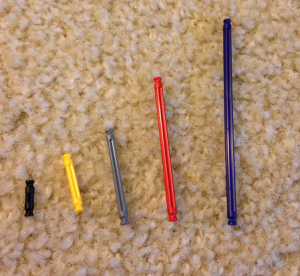One of the basic shapes that will be used by children to construct a variety of objects is an isosceles right triangle.  You can see an example of a bicycle built from K’nex in the picture below.  Notice the two isosceles right triangles making the frame of the bike.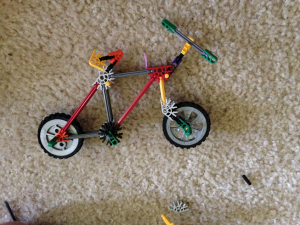Problem 1 (just uses Pythagorean Theorem)

If the length of the gray bars which will form the legs of the isosceles right triangle is 4.1 cm and if it is 0.6 cm from the end of the bar to the center of the connector (the vertex of the triangle), how long should the red bars be made which will form the hypotenuse of the triangle.  See the picture below for reference.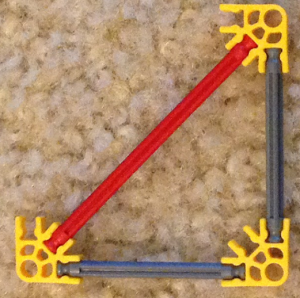Problem 2 (uses Pythagorean theorem and function composition)

Let x be the length of the legs of the smallest isosceles right triangle it will be possible to make with your construction toy.  These legs will consist of a black stick with a connector on each end.  The distance from the end of the stick to the center of the connector (the vertex of the triangle) is 0.6 cm.  The hypotenuse of this smallest triangle will consist of a yellow stick attached to the connectors.  See the picture below.  Give all answers in a complete sentence with units.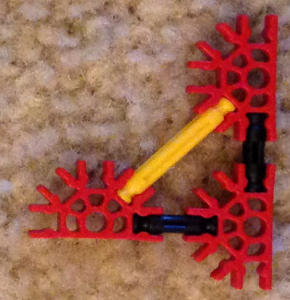a)  (First lets try to design the set of toy’s the easy way.) The easiest thing to do would be to pick integer lengths for the lengths of the sticks.  Suppose the set of toys is manufactured so that the black sticks has length 2 and the yellow sticks have length 3.  Can an isosceles right triangle be built from such a set of toys?  Why or why not?

b) (Ok, so the easy way didn’t work.  For the rest of the worksheet, lets try being more careful.) What is the length of the black stick?  (Give a formula in terms of x).  For the rest of this problem, it will be important to keep track of whether we are discussing the length of a stick or the length of the whole side of the triangle.  You might find it useful to record your answers in the table included at the end of the worksheet so that you can refer back to them as you work.

c)  Give a function f(x) for the length of the hypotenuse of a triangle whose legs have length x.

d) Suppose you decide to make the black sticks 1.4 cm long.  What is the length of the legs of the isosceles right triangle that can be made with them as shown in the picture?  What is the length of the hypotenuse of that triangle?

e) Still supposing you decide to make the black sticks 1.4 cm long, what is the length of the hypotenuse of the triangle described in the previous problem? Compute the length of the hypotenuse in two ways and make sure you get the same answer both times.  %using f(x) and usin the Pythagorean theorem directly.

f)  Go back to assuming the length of the legs (built from black sticks) of the smallest triangle is the unknown x.  Recall that the length of the hypotenuse (built from a yellow stick) of this triangle is given by f(x).  Suppose you wanted to allow bigger isosceles right triangles to be built.  If the legs are built from yellow sticks, use the Pythagorean theorem to give a formula, in terms of x, for the length of the hypotenuse of the triangle with legs made from yellow sticks.  We will use gray sticks for this larger hypotenuse.  The following picture shows the whole set of possible isosceles right triangles.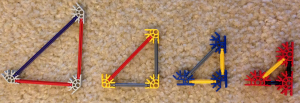g)  Compute \$f \circ f (x)\$ and compare your answer to the formula in the previous problem.  (You may need to do some simplification in order to compare well.)  Explain why any similarities you noticed make sense.

h) Suppose you want to make a third size triangle, this one with legs formed from the gray sticks and a hypotenuse which we will make red.  Does \$ f \circ f \circ f (x) \$ have any bearing on this problem, if so what?

i) Go back to assuming the black sticks will be 1.4 cm long?  How long will the gray sticks be?  How long will the red sticks be?

j)  Still assuming the black sticks are 1.4 cm long, how long will the hypotenuse be of the triangle whose legs are made from red sticks?  How long should we make the purple sticks we will use to form this hypotenuse?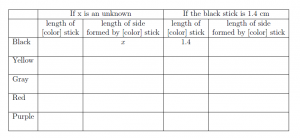Dr. Dawn's blog